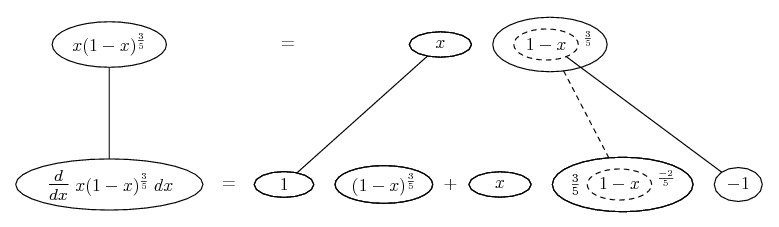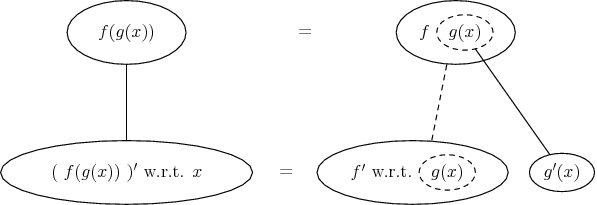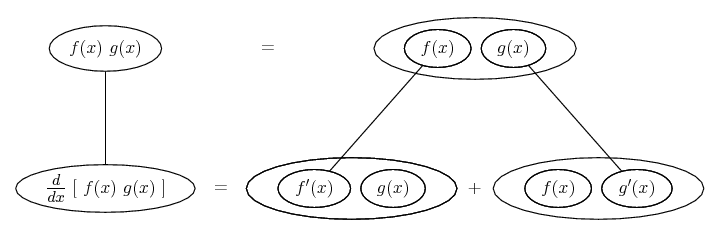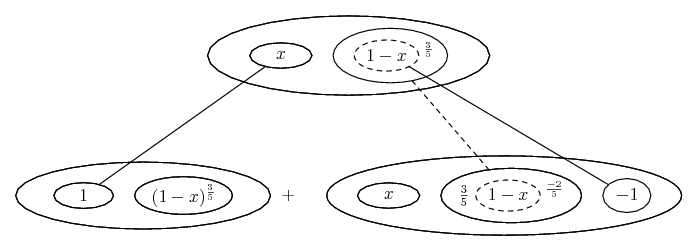# Thread: Quick derivative question

1. ## Quick derivative question

I was wondering if any kind soul would let me know if I needed to use the chain rule to take the derivative of this function.

$\displaystyle f(x)=x(1-x)^3/5$

BTW, I could not get the rational exponent to show up properly. the $\displaystyle (1-x)$ portion is raised to the 3/5ths power.

Thanks!

2.Originally Posted by rust1477I was wondering if any kind soul would let me know if I needed to use the chain rule to take the derivative of this function.

$\displaystyle f(x)=x(1-x)^3/5$

BTW, I could not get the rational exponent to show up properly. the $\displaystyle (1-x)$ portion is raised to the 3/5ths power.

Thanks!
is this your problem
$\displaystyle f(x)=x(1-x)^{3/5}$

?

use product rule
the derivative of $\displaystyle (1-x)^{3/5}$ is
$\displaystyle \frac{3}{5}(1-x)^{\frac{-2}{5}}(-1)$

3.Originally Posted by GaloisTheory1is this your problem
$\displaystyle f(x)=x(1-x)^{3/5}$

?

use product rule
the derivative of $\displaystyle (1-x)^{3/5}$ is
$\displaystyle \frac{3}{5}(1-x)^{\frac{-2}{5}}(-1)$

the function at the bottom of your post is the correct one.
Just to be clear though, I would not have to use the chain rule to take the derivative of $\displaystyle x(1-x)^{3/5}$ correct?

4.Originally Posted by rust1477the function at the bottom of your post is the correct one.
Just to be clear though, I would not have to use the chain rule to take the derivative of $\displaystyle x(1-x)^{3/5}$ correct?
here you can't use chain rule because its a function of x times a function of x so you have to use product rule

5. Gotchta, thanks so much!

Is there any way you could elaborate on what you did to arrive at the final derivative?

6. Just in case a picture helps...So, strictly speaking the chain rule operates, but the inner function is very simple and its derivative just the minus one. For reference, the chain rule generally...... in this case wrapped inside the product rule...... more visible here if we enclose some of the balloons in some more...Hope this helps, or doesn't further confuse.

Don't integrate - balloontegrate!

Balloon Calculus: worked examples from past papers

#### Search Tags

derivative, question, quick